Electron. J. Diff. Equ., Vol. 2012 (2012), No. 215, pp. 1-27.

### Positive solutions of fractional differential equations with derivative terms Cuiping Cheng, Zhaosheng Feng, Youhui Su

Abstract:
In this article, we are concerned with the existence of positive solutions for nonlinear fractional differential equation whose nonlinearity contains the first-order derivative,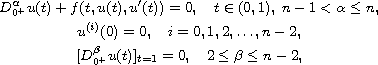where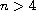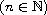,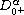is the standard Riemann-Liouville fractional derivative of orderand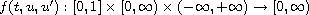satisfies the Caratheodory type condition. Sufficient conditions are obtained for the existence of at least one or two positive solutions by using the nonlinear alternative of the Leray-Schauder type and Krasnosel'skii's fixed point theorem. In addition, several other sufficient conditions are established for the existence of at least triple, n or 2n-1 positive solutions. Two examples are given to illustrate our theoretical results.

Submitted August 15, 2012. Published November 29, 2012.
Math Subject Classifications: 34A08, 34B18, 34K37.
Key Words: Positive solution; equicontinuity; fractional differential equation; fixed point theorem; Caratheodory type condition.

Show me the PDF file (350 KB), TEX file, and other files for this article.Cuiping Cheng Department of Applied Mathematics Hangzhou Dianzi University Hangzhou 310018, China email: chengcp0611@163.com Zhaosheng Feng Department of Mathematics University of Texas-Pan American Edinburg, TX 78539, USA email: zsfeng@utpa.edu; fax: (956) 665-5091 Youhui Su Department of Mathematics Xuzhou Institute of Technology, Xuzhou 221116, China Department of Mathematics, Indiana University Bloomington, IN 47405, USA email: youhsu@indiana.edu